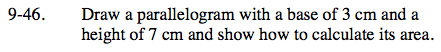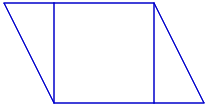Home > ACC7 > Chapter cc29 > Lesson cc29.1.3 > Problem9-46

9-46.

Draw a parallelogram with a base of 3 cm and a height of 7 cm and show how to calculate its area. Homework Help ✎An example of a parallelogram is given below.
It is divided into triangles and a square.
You can use this strategy to find the area of a parallelogram.